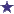# What is the percentage strength of this NEW, Efudex-like compound?New Efudex-Like Compound: Percentage Strength?

Efudex cream is 5% flurouracil and is available in a 25 gram tube. What would be the new percentage strength if in the compounding lab, you added an additional 2 grams of flurouracil powder to the whole tube of efudex cream, making a new compounded product?

### Comments for What is the percentage strength of this NEW, Efudex-like compound?

Average RatingRatingNew, Compounded Product: 8.3% percentage strength of fluroouracil by: David First, we add the 2 grams of the product to the 25 grams of Efudex to get the total weight... 2gms + 25 grams = 27 total grams. Next, we figure how much of 25 grams is 5%? 0.05 X 25 = 1.25 grams Now we add 1.25 grams and 2 grams of the efudex product together... 1.25grams + 2 grams = 3.25 grams Next, to get the new percentage strength, we divide the new total volume by the actual concentrate... 27 grams divided by 3.25 grams = 8.3% percentage strength Thank you for the question!

 RatingPercentage Strength Question by: David Shedd You've stumped me. Any visitors out there who would care to contribute the answer to this question?